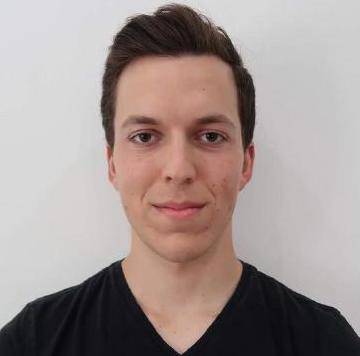# Causal ModelsSt John Aug 07, 2020 · 4 mins readLast time we discussed and motivated the need for a modern theory of causal inference. We developed some of the basic principles necessary to develop this theory, but we have yet to strictly define what exactly a causal model entails. In this ‘episode’ we’ll briefly introduce the notion of a structural causal model and give some examples and implications of how this can be used to make causal statements and assessments. Note, we’ll be discussing SCMs for the case of two variables only. In future discussions we’ll formulate a rigorous and general, set theoretic extension of these definitions. For now, just keep in mind that this theory generalises. Let’s begin!

## Causal Models

Structural Causal Model: An SCM $\mathbb{C}$ with graph $C \rightarrow E$ consists of two assignments $C:=N_{C}$ $E:=f_{E}\left(C, N_{E}\right)$ where the noise terms, $N_E$ and $N_C$ are independent of each other. Here $C$ is the cause and $E$ is the effect. Given knowledge of the function $f_E$, we can sample from the SCM by first evaluating $C$ and then $E$. The SCM thus gives a joint distribution $P_{C,E}$ over both $C$ and then $E$.

We can play around with this distribution in R.

1
2
3
4
5
6
7
8
set.seed(1)

# Generate 500 random samples from the SCM discussed above
C <- rnorm(500)
E <- 4*C + rnorm(500)

(statistics <- c(mean(E),var(E)))
# My result:   0.4463598 17.14897886


## Interventions

A major motivation for the the development of causal inference theory is the idea of intervening in a system, as discussed in previously. Intervening induces a new distribution, distinct from the observational distribution. There are two distinct ways we can intervene on the system:

1. Hard interventions involve setting the value of one of the causal nodes. For example, setting $E:= 4$ is written $do(E:=4)$ with interventional distribution now $P_C^{do(E:=4)}$.
2. Soft interventions involve modifying the assignment operation of the SCM. Consider $do(E:=g_E(C) + \tilde{N}_E )$.

Let’s modify our previous model in R with a hard intervention of $do(E:=4)$:

1
2
3
4
5
C <- rep(4,500)
E <- 4*C + rnorm(500)

(statistics <- c(mean(E),var(E)))
# My result:  16.022644  1.023999


Interestingly, the asymmetry between the cause and effect relationship can be written as a statement of independence. Consider that changing the cause necessarily changes the effect, but changing the effect does not cause a change in the cause. By intervening on $E$ by randomising, we can effectively make $C$ and $E$ independent. The interventional distribution is then $P_{C,E}^{\mathbb{C};do(E:=\tilde{N}_E)}.$

Once again, we can generate a sample from the distribution using R:

1
2
3
4
5
6
C <- rnorm(500)
E <- rnorm(500)

# Check the correlation p-value
(correlation <- corr.test(C,E)\$p.value)
# My result:  0.3575716


## Counterfactuals

Counterfactual reasoning is regarded by many to be the cornerstone of human intelligence. This, according to Pearl’s ‘ladder of causation’, is a level 3 task. A counterfactual is, as it sounds, an event that is “counter to the facts”. In other words, it is a “what if?” type question in which the agent reasoning imagines a situation and outcome given a certain event did or did not occur, even though we already have observed a different outcome. For example, given Newton observed an apple falling from a tree and heard a distinct thud, he could ask what would have happened if the apple had not fallen. This is counterfactual reasoning.If we want machines to exhibit human level intelligence, we need to find a way to encode counterfactual reasoning.

Structural causal models and causal inference address the lack of counterfactual structure in conventional statistical approaches. Formally, changing the noise distributions of an SCM allows us to ask and answer counterfactual questions. When Newton saw the apple fall and heard the noise, he gained information about the system. This new information about the system can be used to retrospectively reason about what would have happened even in a different scenario, because he now knows more about how the system behaves.

## Next Up

In this article we developed some of the more formal theory of structural causal models and discussed some of the tools these models afford us. In the next episode of the series we’ll discuss how we can start learning the underlying structure from data - a seemingly impossible task. To do this, we’ll need to discuss some assumptions and restrictions on the type of models we can use. It’s going to be a good one!

### Resources

This series of articles is largely based on the great work by Jonas Peters, among others:##### Written by St John
Author of the Asking Why Blog - a personal blog and website with everything I find interesting.